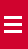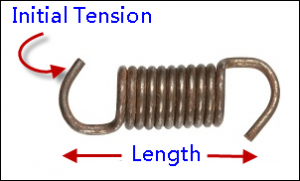# What is an initial tension?

Initial tension is the tension that has already accumulated between coils of extension springs. This tension helps keep the coils together. The initial tension is released, when an extension spring is extended to a certain point, where you can see gap between the two coils.### How to calculate initial tension?

For example: You are working with an extension spring with following specifications:

Rate (R) = 5 pounds per inch

Working Load (L) = 10 pounds per inch

Distance Traveled (T) = 1 inches

The initial tension is calculated as IT= L-RT

IT = 10-5(1)

IT= 5lbf